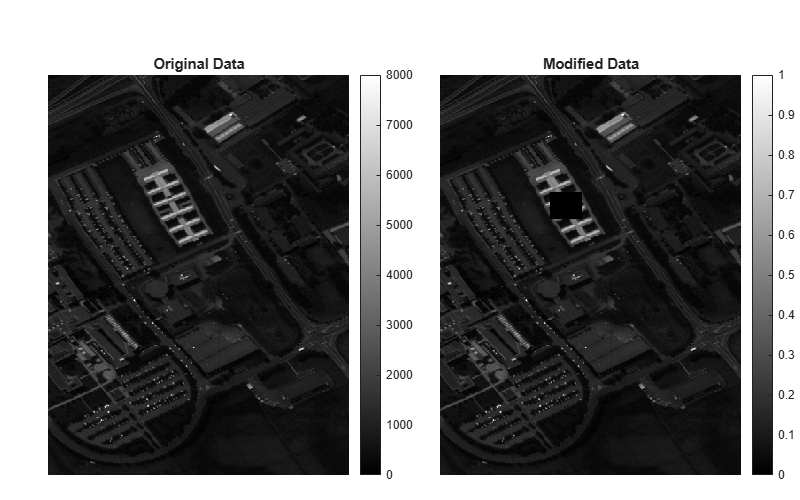# assignData

Assign new data to hyperspectral data cube

## Syntax

``newhcube = assignData(hcube,row,column,band,data)``

## Description

example

````newhcube = assignData(hcube,row,column,band,data)` assigns the specified `data` to a hyperspectral data cube. The function reads the data cube stored in the `hypercube` object `hcube`, assigns the new data to the spectral bands `band` at the locations specified by `row` and `column`, and returns a new `hypercube` object. NoteThis function requires the Image Processing Toolbox™ Hyperspectral Imaging Library. You can install the Image Processing Toolbox Hyperspectral Imaging Library from Add-On Explorer. For more information about installing add-ons, see Get and Manage Add-Ons. ```

## Examples

collapse all

Read hyperspectral data from an ENVI format file.

`hcube = hypercube('paviaU.dat');`

Normalize the reflectance values to the range [0, 1].

`data = rescale(hcube.DataCube);`

Assign the normalized reflectance values to the data cube.

`newhcube = assignData(hcube,':',':',':',data);`

Specify the row and column indices of a region of interest (ROI). Assign all indices within the ROI a value of zero.

```row = 180:220; column = 125:160; newhcube = assignData(newhcube,row,column,':',0);```

Display the original and the modified versions of a spectral band.

```fig = figure('Position',[0 0 800 500]); axes1 = axes('Parent',fig,'Position',[0.06 0.05 0.45 0.8]); imagesc(hcube.DataCube(:,:,10),'Parent',axes1); title('Original Data') colorbar axis off axes2 = axes('Parent',fig,'Position',[0.55 0.05 0.45 0.8]); imagesc(newhcube.DataCube(:,:,10),'Parent',axes2); title('Modified Data') colorbar axis off colormap gray ```## Input Arguments

collapse all

Input hyperspectral data, specified as a `hypercube` object. The `DataCube` property of the `hypercube` object contains the hyperspectral data cube.

Row indices of the data cube, specified as `':'`, a positive integer, or a vector of positive integers.

• To select all the rows in the data cube, use ` ':'`.

• To select a particular row or rows, specify the row index as a positive integer or vector of positive integers respectively. If the data cube is of size M-by-N-by-C, the specified row index values must all be less than or equal to M. To specify a range of row indices, or indices at a regular interval, use the `colon` operator. For example, `row = 1:10`.

Data Types: `single` | `double` | `int8` | `int16` | `int32` | `int64` | `uint8` | `uint16` | `uint32` | `uint64` | `char` | `string`

Column indices of the data cube, specified as `':'`, a positive integer, or a vector of positive integers.

• To select all the columns in the data cube, use ``` ':'```.

• To select a particular column or columns, specify the column index as a positive integer or vector of positive integers respectively. If the data cube is of size M-by-N-by-C, the specified column index values must all be less than or equal to N. To specify a range of column indices, or indices at a regular interval, use the `colon` operator. For example, ```column = 1:10```.

Data Types: `single` | `double` | `int8` | `int16` | `int32` | `int64` | `uint8` | `uint16` | `uint32` | `uint64` | `char` | `string`

Spectral band numbers, specified as `':'`, a positive integer or a vector of positive integers.

• To select all the bands in the data cube, use `':'`.

• To select a particular band or bands, specify the band number as a positive integer or vector of positive integers respectively. If the data cube is of size M-by-N-by-C, the specified band number values must all be less than or equal to C. To specify a range of band numbers or numbers at a regular interval, use the `colon` operator. For example, ```band = 1:10```.

Data Types: `single` | `double` | `int8` | `int16` | `int32` | `int64` | `uint8` | `uint16` | `uint32` | `uint64` | `char` | `string`

Values to assign, specified as a scalar, vector, matrix, or 3-D array depending on the values of the `row`, `column`, and `band` inputs.

If `row` isIf `column` isIf `band` is`data` must be
scalarscalarscalarscalar
M- element vectorscalarscalarM- element row vector or M-by-1 matrix or M-by-1-by-1 array
scalarN-element vectorscalarN- element column vector or 1-by-N matrix or 1-by-N-by-1 array
scalarscalarC-element vector1-by-1-by-C array
M- element vectorN-element vectorscalarM-by-N matrix or M-by-N-by-1 array
M- element vectorN-element vectorC-element vectorM-by-N-by-C array

Data Types: `single` | `double` | `int8` | `int16` | `int32` | `int64` | `uint8` | `uint16` | `uint32` | `uint64`

## Output Arguments

collapse all

Output hyperspectral data, returned as a `hypercube` object.